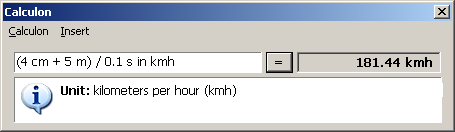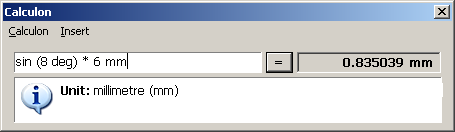# Calculon

Current version 1.0-alpha1

Some of the key features:

• Lean and mean — designed for keyboard use, minimal UI kludge.
• Evaluates expressions — makes it easier to fix typos and minimizes the need to retype long calculations. Just type in the full equation and press enter.
• Unit, currency and other conversions — you can mix inches, metres, nanoseconds, kelvins, radians and hectares with no need converting measures to a common base.
• Extendable — units, functions, conversions and constants can be added and customized.

Calculon is a free2, tiny desktop calculator for Microsoft Windows (98, 2000, XP, Vista) that calculates by evaluating expressions instead of emulating a traditional desk calculator. Calculon is extremely extendable which means you can use it to solve practical problems such as “How long will it take to download this file?” and so on3.

The ideal usage pattern for Calculon goes a bit like this: launch Calculon with a hotkey, type or paste in the calculation, press enter and copy the result.

Calculon ships with predefined constants, functions and units but anyone can easily add the functionality they need. If you are a historian, you can add ancient measures or if like to cook, you can make Calculon convert between teaspoons and gallons if that is what you need.### Known bugs in 1.0-alpha

Random numbers are generated every time Calculon evaluates an expression and also when it looks for the unit of the expression. This means if you are using a ternary operator, it will lead into random behavior.

`(rnd > 0.5) ? 1 m : 3 h`

The above will return any of the following: 1 m, 3 h, 3 m, 1 h. This is because the expression (rnd > 0.5) is evaluated first for the number part and for a second time for the unit.

1. Calculon is in a working preview state. Some features are missing or unfinished.
2. Calculon is freeware.
3. See the quick start page for more practical examples